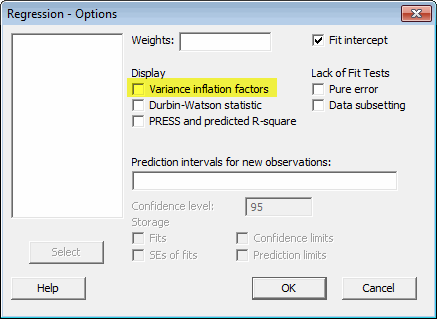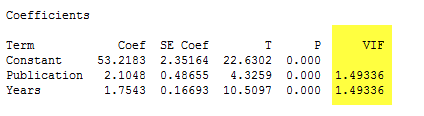Topics: Regression Analysis

In regression analysis, we look at the correlations between one or more input variables, or factors, and a response. We might look at how baking time and temperature relate to the hardness of a piece of plastic, or how educational levels and the region of one's birth relate to annual income. The number of potential factors you might include in a regression model is limited only by your imagination...and your capacity to actually gather the data you imagine.

But before throwing data about every potential predictor under the sun into your regression model, remember a thing called multicollinearity. With regression, as with so many things in life, there comes a point where adding more is not better. In fact, sometimes not only does adding "more" factors to a regression model fail to make things clearer, it actually makes things harder to understand!

## What Is Multicollinearity and Why Should I Care?In regression, "multicollinearity" refers to predictors that are correlated with other predictors.  Multicollinearity occurs when your model includes multiple factors that are correlated not just to your response variable, but also to each other. In other words, it results when you have factors that are a bit redundant.

You can think about it in terms of a football game: If one player tackles the opposing quarterback, it's easy to give credit for the sack where credit's due. But if three players are tackling the quarterback simultaneously, it's much more difficult to determine which of the three makes the biggest contribution to the sack.

Not that into football?  All right, try this analogy instead: You go to see a rock and roll band with two great guitar players. You're eager to see which one plays best. But on stage, they're both playing furious leads at the same time!  When they're both playing loud and fast, how can you tell which guitarist has the biggest effect on the sound?  Even though they aren't playing the same notes, what they're doing is so similar it's difficult to tell one from the other.

That's the problem with multicollinearity.

Multicollinearity increases the standard errors of the coefficients. Increased standard errors in turn means that coefficients for some independent variables may be found not to be significantly different from 0. In other words, by overinflating the standard errors, multicollinearity makes some variables statistically insignificant when they should be significant. Without multicollinearity (and thus, with lower standard errors), those coefficients might be significant.

## Warning Signs of Multicollinearity

A little bit of multicollinearity isn't necessarily a huge problem: extending the rock band analogy, if one guitar player is louder than the other, you can easily tell them apart. But severe multicollinearity is a major problem, because it increases the variance of the regression coefficients, making them unstable. The more variance they have, the more difficult it is to interpret the coefficients.

So, how do you know if you need to be concerned about multicollinearity in your regression model? Here are some things to watch for:

• A regression coefficient is not significant even though, theoretically, that variable should be highly correlated with Y.
• When you add or delete an X variable, the regression coefficients change dramatically.
• You see a negative regression coefficient when your response should increase along with X.
• You see a positive regression coefficient when the response should decrease as X increases.
• Your X variables have high pairwise correlations.

One way to measure multicollinearity is the variance inflation factor (VIF), which assesses how much the variance of an estimated regression coefficient increases if your predictors are correlated.  If no factors are correlated, the VIFs will all be 1.

To have Minitab Statistical Software calculate and display the VIF for your regression coefficients, just select it in the "Options" dialog when you perform your analysis.With Display VIF selected as an option, Minitab will provide a table of coefficients as part of its output.  Here's an example involving some data looking at the relationship between researcher salary, publications, and years of employment:If the VIF is equal to 1 there is no multicollinearity among factors, but if the VIF is greater than 1, the predictors may be moderately correlated. The output above shows that the VIF for the Publication and Years factors are about 1.5, which indicates some correlation, but not enough to be overly concerned about. A VIF between 5 and 10 indicates high correlation that may be problematic. And if the VIF goes above 10, you can assume that the regression coefficients are poorly estimated due to multicollinearity.

You'll want to do something about that.

## How Can I Deal With Multicollinearity?

If multicollinearity is a problem in your model -- if the VIF for a factor is near or above 5 -- the solution may be relatively simple. Try one of these:

• Remove highly correlated predictors from the model.  If you have two or more factors with a high VIF, remove one from the model. Because they supply redundant information, removing one of the correlated factors usually doesn't drastically reduce the R-squared.  Consider using stepwise regression, best subsets regression, or specialized knowledge of the data set to remove these variables. Select the model that has the highest R-squared value.

• Use Partial Least Squares Regression (PLS) or Principal Components Analysis, regression methods that cut the number of predictors to a smaller set of uncorrelated components.

With Minitab Statistical Software, it's easy to use the tools available in Stat > Regression menu to quickly test different regression models to find the best one. If you're not using it, we invite you to try Minitab for free for 30 days.

Have you ever run into issues with multicollinearity? How did you solve the problem?